# Introduction Chemistry Matter Anything that has mass and

• Slides: 19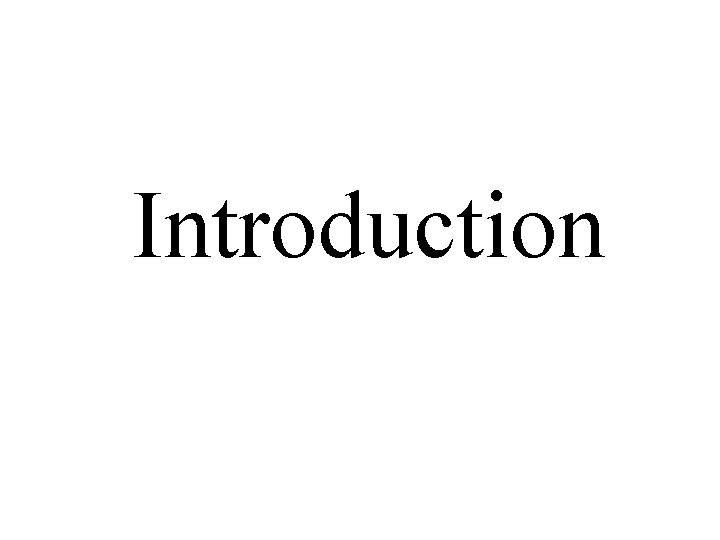IntroductionChemistry • Matter: Anything that has mass and occupies space • Chemistry: The science that studies the structure of matter and its transformationsPure Substances and Mixtures • A pure substance: A substance with a constant fixed composition and distinct properties (e. g. , pure water). • A mixture: A combination of two or more pure substances in which each substance maintains its own identity. a) Homogeneous mixture: e. g. , a solution of salt (Na. Cl) in water b) Heterogeneous mixture: e. g. , a mixture oil and water • We can separate the constituents of a solution by physical methods.Pure Substances and MixturesElements and Compounds • Element: Substance that can not be decomposed into simpler substances by chemical methods • Compound: Substance formed of atoms of two or more elements chemically bound in defined proportions. • Compounds can only be separated into their constituents by chemical methods.Physical and Chemical Properties of Matter • Physical Property: A property that can be measured or observed without altering the composition or nature of the substance • Chemical Property: A property that can only be measured or observed by altering the composition or nature of the sustance • Extensive Property: Depends on the quantity of matter being studied • Intensive Property: Does not depend on the quantity of matter being studiedMeasurements • A number that is not followed by a unit does not mean anything • International System (SI): length: meter (m) mass: kilogram (kg) time: second (s) flow of electric current: ampere (A) temperature: Kelvin (K) quantity of matter: mole (mol) luminous intensity: candela (cd) • Prefixes: Mega (M): 106 Kilo (k): 103 Deci (d): 10 -1 Centi (c): 10 -2 Milli (m): 10 -3Mass and Weight • Mass: Measure of the quantity of matter that constitutes an object • Weight: force that gravity exerts on an object • The kilogram (kg) is the SI unit, but we mainly use the gram (g): 1 kg = 1000 g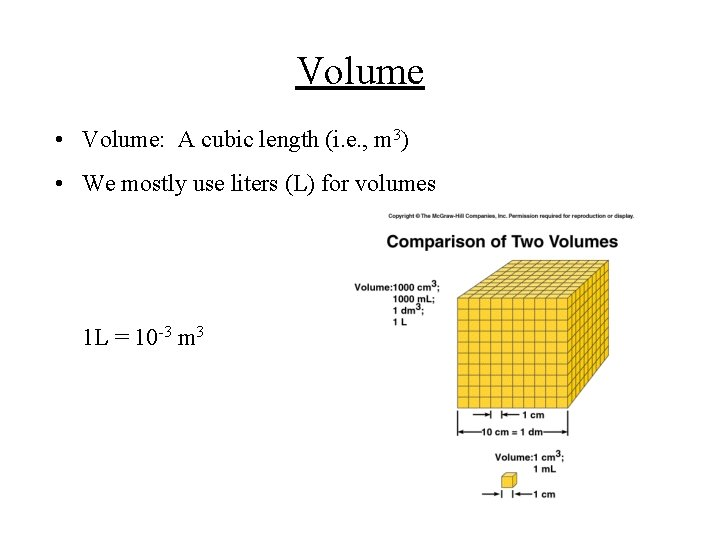Volume • Volume: A cubic length (i. e. , m 3) • We mostly use liters (L) for volumes 1 L = 10 -3 m 3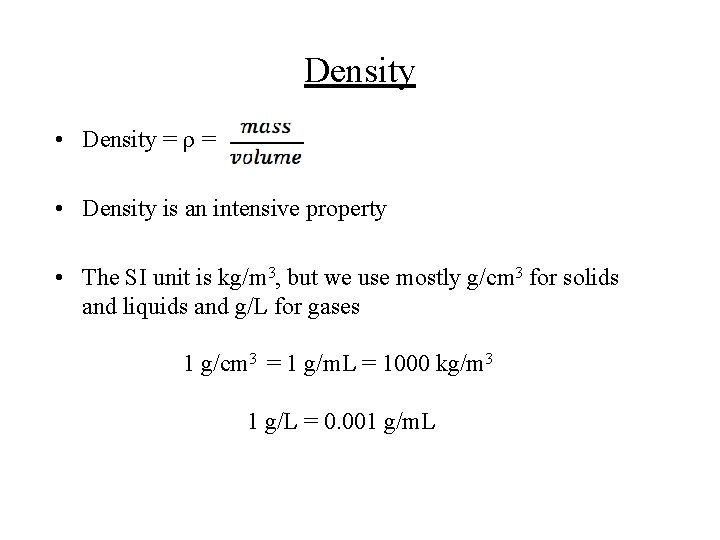Density • Density = r = • Density is an intensive property • The SI unit is kg/m 3, but we use mostly g/cm 3 for solids and liquids and g/L for gases 1 g/cm 3 = 1 g/m. L = 1000 kg/m 3 1 g/L = 0. 001 g/m. LTemperature • The SI unit is Kelvin (K) • The absolute zero of temperature is 0 K or -273. 15 o. C • The freezing point of water is 273. 15 K or 0 o. C • The boiling point of water is 373. 15 K or 100 o. C • Express the temperature in K in your calculations!!!Numerical Operations • Scientific Notation: The form N x 10 n eg. ; 6. 022 045 x 1023 instead of 602 204 500 000 000 000 N=6. 022 045 and n=23 • Significant Digits: Numbers that have significance in the calculation or measurement of a quantity. • e. g. ; if a scale is precise to ± 0. 1 kg, it makes no sense to say that an object has a mass of 6. 73 kg; rather, we say that the object weighs (6. 7± 0. 1) kgSignificant Digits: the Rules • Any non-zero digit is significant: e. g. ; 6. 732 kg has 4 significant digits • A zero placed between two non-zero digits is significant: e. g. ; 6. 0061 kg has 5 significant digits • Zeroes placed to the left of the first non-zero are not significant: e. g. ; 0. 0502 kg has 3 significant digitsSignificant Digits: the Rules • If the number is greater than 1, all the zeroes to the right of the decimal are significant: e. g. ; 6. 000 kg has 4 significant digits • Without scientific notation, it is sometimes ambiguous whether the zeroes to the right of the last non-zero are significant: e. g. ; 4500 kg could be 4. 5 x 103, 4. 50 x 103, or 4. 500 x 103 kg; i. e. , we could have 2, 3, or 4 significant digitsSignificant Digits: the Rules • An integer with perfect precision has an infinite number of significant digits: e. g. ; If we are finding the average of three tests, we have by definition 3. 000000… tests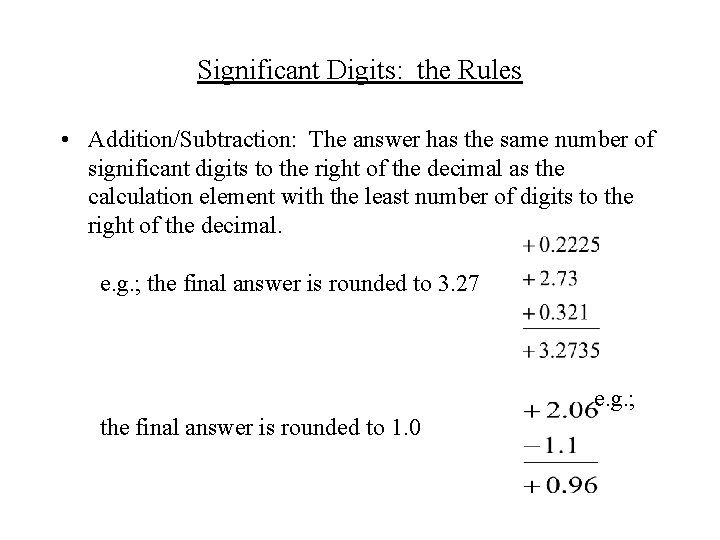Significant Digits: the Rules • Addition/Subtraction: The answer has the same number of significant digits to the right of the decimal as the calculation element with the least number of digits to the right of the decimal. e. g. ; the final answer is rounded to 3. 27 e. g. ; the final answer is rounded to 1. 0Significant Digits: the Rules • Multiplication/Division: The answer has the same number of significant digits as the calculation element with the least number of significant digits. e. g. ; 2. 2 x 3. 7845 = 8. 32590 is rounded to 8. 3 e. g. ; 3. 76 / 4. 236 = 0. 8876298… is rounded to 0. 888 e. g. ; (2. 27 x 7. 324) / 3. 3 = 5. 0380… is rounded to 5. 0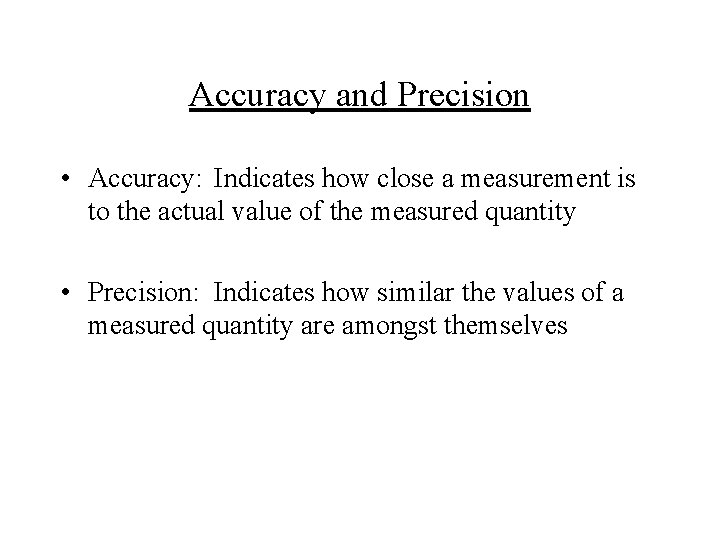Accuracy and Precision • Accuracy: Indicates how close a measurement is to the actual value of the measured quantity • Precision: Indicates how similar the values of a measured quantity are amongst themselvesAccuracy and Precision • (a): accurate and precise • (b): inaccurate, but precise • (c): inaccurate, imprecise • Exact measurements are usually precise, but a systematic error can give precise measurements that are not accurate